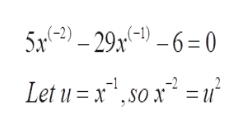Solve the equation after making an appropriate substitution.5x^(-2)-29x^(-1)-6=0Determine the appropriate substitution using the new variable "u."Next, write the new quadratic equation using the variable "u."Then, solve the new quadratic equation for the variable "u."Finally, give the solution set to the original equation.

Question

Solve the equation after making an appropriate substitution.

5x^(-2)-29x^(-1)-6=0

Determine the appropriate substitution using the new variable "u."

Next, write the new quadratic equation using the variable "u."

Then, solve the new quadratic equation for the variable "u."

Finally, give the solution set to the original equation.

Step 1

To solve the given equation : to find the value of x by making a suitable substitution to reduce to a quadratic equation

Step 2

The given equation is actually a quadratic equation in the unknown 1/x. So, set u =1/x and solve it

Step 3

Effect of subst...help_outlineImage Transcriptionclose5-2)-29x-1)-6= 0 Let u = x1 ,so x2 = u fullscreen

Want to see the full answer?

See Solution

Want to see this answer and more?

Our solutions are written by experts, many with advanced degrees, and available 24/7

See Solution
Tagged in

Math